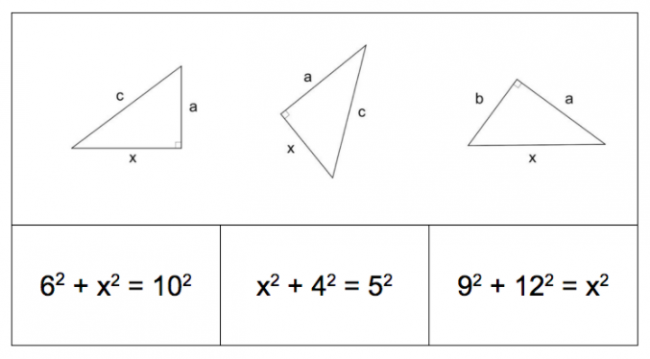# Pythagorean Theorem: What is unknown?

Preview:This task focuses students on how structural differences in which side is unknown in a right triangle lead to different equations to find the missing side. For this task, choose one of the triangles or one of the equations for students to create.

Connecting Representations
Big Idea:
Grade 8: The relationship between surface area and volume of 3D objects can be used to describe, measure, and compare spatial relationships.
Content:
modelling the Pythagorean theorem, finding a missing side of a right triangle
Curricular Competency:
Reasoning and analyzing: Develop mental math strategies and abilities to make sense of quantities
Understanding and solving: Develop, demonstrate, and apply mathematical understanding through play, inquiry, and problem solving
Understanding and solving: Visualize to explore mathematical concepts
Understanding and solving: Develop and use multiple strategies to engage in problem solving
Communicating and representing: Communicate mathematical thinking in many ways
Communicating and representing: Use mathematical vocabulary and language to contribute to mathematical discussions
Communicating and representing: Explain and justify mathematical ideas and decisions
Communicating and representing: Represent mathematical ideas in concrete, pictorial, and symbolic forms
Connecting and reflecting: Reflect on mathematical thinking